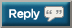# Thread: Hey guys, let's pound out a million pull-ups by end of 2014 (3.0)

1. 4x12, 1x2

38,590 + 50 = 38,6402. My 5 min challenge for the O35 Chin-up Project yesterday yielded 66, now on to weighted ones38,640 + 66 = 38,7063. My 5 min challenge for the O35 Chin-up Project yesterday yielded 66, now on to weighted ones38,640 + 66 = 38,706
38,706 + 25 = 38,7314. Pounded out anotha fitty today

38,731+50=38,7815. Pounded out anotha fitty today

38,731+50=38,781
38,781 + 30 = 38,8116. 38,811 + 45 = 38,8567.8.9. 38,929 + 28 = 38,95710. 38,957 + 5 pull ups + 10 weighted pull ups(+25lbs) = 38,97211. 38,957 + 5 pull ups + 10 weighted pull ups(+25lbs) = 38,972

38 972 + 56 = 39 02812. 39,028+65=39,09313.14.15.16. 14 weighted & 14 non

39,290 + 28 = 39,31817. 14 weighted & 14 non

39,290 + 28 = 39,318
39,318 +100 = 39,41818. 34,418+ 34 = 34,45119. 34,451 + 50 = 34,50120. 34,501 + 476 = 34,97721.22. 4x15

35,007 + 60 = 35,06723. 35,067 + 48 = 35,11524.25.26. Somehow it looks like jamalfudge lost my 48, so the correct number now should be:

35,126 + 48 = 35,17427. Somehow it looks like jamalfudge lost my 48, so the correct number now should be:

35,126 + 48 = 35,174
35,174 + 47 = 35,22128. Did total of 25 today.
35,221+25= 35,24629. 35.246 + 25 = 35.27130. 35,271 + 50 = 35,321#### Posting Permissions

• You may not post new threads
• You may not post replies
• You may not post attachments
• You may not edit your posts